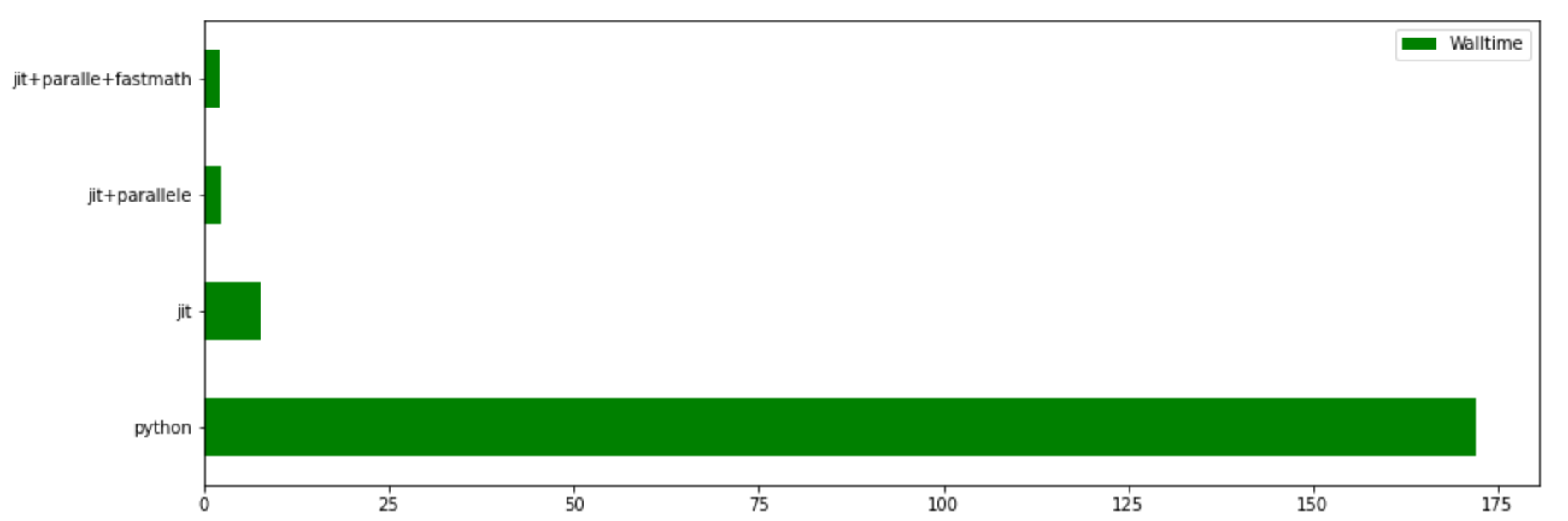# Accelerating Python applications with Numba

## Introduction

Numba accelerates the computation and processing of Python/NumPy code by compiling Python code into native machine code. Numba provides rich decorators for vectorization and paralelization.

## Prerequisites

You will need first to start an interactive job on MeluXina, in order to have access to the software environment available on the compute nodes. Once the job has started, load a Python profile using the `module load` command. Note that if no version is specified the latest available will be activated. If you have an existing set of packages, libraries or code that require a specific Python version you will need to load it explicitly.

``````module load Python
``````

For this example we will require numpy and numba, which are part of the `SciPy-bundle` package on MeluXina.

``````module load SciPy-bundle
``````

## Automatic Multi-threading and Paralleling in Loops/Iterations

NumPy array expressions have a significant amount of implied parallelism, as operations are broadcast independently over the input elements. ParallelAccelerator can identify this parallelism and automatically distribute it over several threads. All we need is to enable the parallelization pass with `@jit`, `@parallel=True`, `@prange` and `@fastmath=True` annotations.

For example, we might want to run many Monte Carlo trials in a row with 4 different implementations:

• Pure Python code
``````def monte_carlo_pi(nsamples):
acc = 0
for i in range(nsamples):
x = random.random()
y = random.random()
if (x**2 + y**2) < 1.0:
acc += 1
return 4.0 * acc / nsamples
``````
• Serial version
``````@jit(nopython=True)
def monte_carlo_pi_serial(nsamples):
acc = 0
for i in range(nsamples):
x = random.random()
y = random.random()
if (x**2 + y**2) < 1.0:
acc += 1
return 4.0 * acc / nsamples
``````
• Parallel version
``````@jit(nopython=True, parallel=True)
def monte_carlo_pi_parallel(nsamples):
acc = 0
# Only change is here
for i in numba.prange(nsamples):
x = random.random()
y = random.random()
if (x**2 + y**2) < 1.0:
acc += 1
return 4.0 * acc / nsamples
``````
• Parallel + fastmath optimization version
``````@jit(nopython=True, parallel=True, fastmath=True)
def monte_carlo_pi_parallel(nsamples):
acc = 0
# Only change is here
for i in numba.prange(nsamples):
x = random.random()
y = random.random()
if (x**2 + y**2) < 1.0:
acc += 1
return 4.0 * acc / nsamples
``````

Let's see how fast these 4 implementations are:

``````%time monte_carlo_pi(int(4e8))
%time monte_carlo_pi_serial(int(4e8))
%time monte_carlo_pi_parallel(int(4e8))
%time monte_carlo_pi_parallel_fastmath(int(4e8))

CPU times: user 2min 51s, sys: 17 ms, total: 2min 51s
Wall time: 2min 52s
CPU times: user 4.35 s, sys: 3.23 s, total: 7.57 s
Wall time: 7.6 s
CPU times: user 10.3 s, sys: 439 ms, total: 10.8 s
Wall time: 2.3 s
CPU times: user 10.6 s, sys: 14.2 ms, total: 10.6 s
Wall time: 2.06 s
3.14155854
``````

Observation: With a combination of `parallel=True`, `fastmath=True` and `prange` optimizations the code is more than 84 times faster than the initial pure Python implementationFor additional showcases on using numba, see: